# The Risk of Bankruptcy

This entry is part 1 of 4 in the series Risk of Bankruptcy

Investors should be skeptical of history-based models. Constructed by a nerdy-sounding priesthood using esoteric terms such as beta, gamma, sigma and the like, these models tend to look impressive. Too often, though, investors forget to examine the assumptions behind the symbols. Our advice: Beware of geeks bearing formulas.  – Warren E. Buffett.1

Historic growth is usually a risky estimate for future growth. To be able to forecast a company’s future performance you have to make assumptions on the future most likely values and their event space of a large number of variables, and then calculate both the probability of future necessary cash infusions and if they do not materialized – the risk of bankruptcy.

The following calculations are carried out using the Strategy& Risk simulation model. Such simulations can be carried out on all types of enterprises including the financial sector. There are several models in use for predicting bankruptcy and we have in our balance simulation model implemented two;  Altman’s Z-score model and the risk index Z developed by Hannan and Hanweck.

Atman’s Z-score model is based on financial ratios and their relation to bankruptcy found from discriminant analysis.2  The coefficients in the discriminant function has in later studies been revised – the Z’-score and Z’’-score models.

Hannan and Hanweck’s probability of insolvency is based on the likelihood of return to assets being negative and larger then the capital-asset ratio.3 The Z index has been used  to forecast bank insolvency45, but can profitably be used to study large private companies with low return to assets.

We will here take a look at the Z-index and in a later post use the same data to calculate the Z-scores.

The following calculations are based on forecasts, EBITDA and balance simulations – not on historic balance sheet data. The Z-index is defined as:

Z=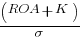where ROA is the pre-tax return on assets, K the ratio of equity to assets, and s the standard deviation of pre-tax ROA. The Z-index give pr unit of standard deviation of ROA the decline in ROA the company can manage before equity is exhausted and becomes insolvent.

We will in the simulation (250 runs) for every year in the 15 year forecast period – both forecast the yearly ROA and K, and use the variance in ROA to estimate s. For every value of Z – assuming a symmetric distribution – we can calculate the perceived probability (upper bound) of insolvency (p) from:

P =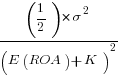where the multiplication by (1/2) reflects the fact that insolvensy occurs only in the left tail of the distribution. The relation of p to Z is inverse one, with higher Z-ratios indicating low probability of insolvency.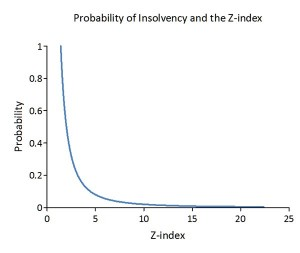Since our simulation cover a 15 year period it is fully possible that multi-period losses, thru decline in K, can wipe out the equity and cause a failure of the company.

In year five of the simulation the situation is as follows, the pre-tax return on assets is low – on average only 1.3% and in 20% of the cases it is zero or negative.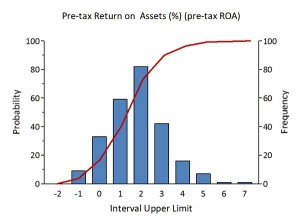However the ratio of equity to assets is high – on average 37% with standard deviation of only 1.2.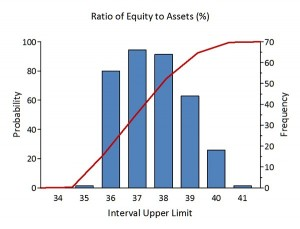The distribution of the corresponding Z-Index values is given in the chart below. It is skewed with a long right tail; the mean is 32 with a minimum value of 16.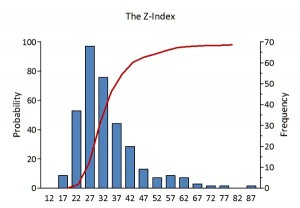From the graph giving the relation between the Z-index and probability of insolvency it is clear that the company’s economic situation is far from being threatened. If we look at the distribution for the probability of insolvency as calculated from the estimated Z-index values this is confirmed having values in the range from 0.1 to 0.3.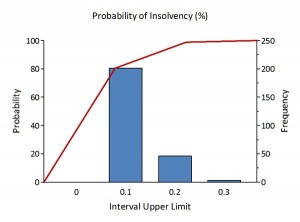Having the probability of insolvency pr year gives us the opportunity to calculate the probability of failure over the forecast period for any chosen strategy.

If it can’t be expressed in figures, it is not science; it is opinion. It has long been known that one horse can run faster than another — but which one? Differences are crucial.6

### References